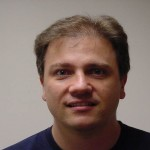# Master of Science in Applied MathematicsConcentration in Dynamical Systems and Chaos

This concentration focuses on interdisciplinary applications of dynamical systems and nonlinear modeling in biology, chemistry, engineering, and physics. Students with interests in modeling and analyzing real life problems through mathematics will benefit from this concentration.

## Preparation

Before entering the program, students must complete the following upper division courses, or equivalent. Students with inadequate undergraduate preparation may be accepted conditionally but will be required to complete courses for removal of the deficiency in the first year of study.

 All of the following Math 237 - Elementary Differential Equations (3 units) Math 330 - Advanced Calculus (3 units) Math 340 - Mathematical Programming (3 units) Math 524 - Linear Algebra (3 units) One of the following Stat 350A - Statistical Methods (3 units) Stat 551A - Probability and Mathematical Statistics (3 units)Ricardo Carretero
Professor
GMCS-577Antonio Palacios
Professor
GMCS-503

## Required Courses (31 units)

The 31 units may include at most 12 units of approved 500-level mathematics courses and at most six units of independent research (Mathematics 797, 798, 799A, 799B). Elective courses from other departments may be approved by the adviser.

 All of the following Math 538 - Discrete Dynamical Systems and Chaos (3 units) Math 636 - Mathematical Modeling (3 units) Math 780 - Seminar in Communicating Mathematical Research (1 unit) [maximum one RP allowed] Math 797 - Research (3 units) Math 799A - Thesis or Project (3 units) One of the following Math 531 - Partial Differential Equations (3 units) Math 537 - Ordinary Differential Equations (3 units) Math 638 - Continuous Dynamical Systems and Chaos (3 units) One of the following Math 630 - Applied Real Analysis (3 units) Math 668 - Applied Fourier Analysis (3 units) One of the following Math 693A - Advanced Numerical Methods: Computational Optimization (3 units) Math 693B - Advanced Numerical Methods: Computational Partial Differential Equations (3 units) 9 units of electives, possibly selected from the following Math 542 - Introduction to Computational Ordinary Differential Equations (3 units) Math 543 - Numerical Matrix Analysis (3 units) Math 635 - Pattern Formation (3 units) Math 639 - Nonlinear Waves (3 units) Math 693A - Advanced Numerical Methods: Computational Optimization (3 units) Math 693B - Advanced Numerical Methods: Computational Partial Differential Equations (3 units) Comp 605/CS 605 - Scientific Computing (3 units)

## Other requirements

Program of study.
The program of study,to include a plan for removal of any conditions on admission, must be approved by the graduate adviser and will include at least 22 units in mathematics.

Thesis.
Students must select Plan A and complete Math 799A (Thesis). Students are advised that a thesis normally takes a year to complete.

### Degree Learning Outcomes

Listed below are the applicable Degree Learning Outcomes (DLOs) for this degree.

• Comprehensive learning. Students will gain fundamental learning and understanding of interdisciplinary concepts and methods of dynamical systems and nonlinear modeling in various fields that include: Biology, Chemistry, Engineering, and Physics. Students will develop the necessary skills to model and analyze real-life problems through analytical and computational mathematical methods at the frontier of applied mathematics and nonlinear science.
Courses: Math 330, Math 337, Math 340, Math 524.
• Original research work. Students will gain experience in conducting original research in dynamical systems, including solving innovative problems that involve phenomena that changes in space and/or time. It is expected for students to develop in-depth understanding of nonlinear mathematical models and numerical methods to solve them.
Courses: Math 531, Math 537, Math 538, Math 636, Math 638.
Electives: Math 542, Math 623, Math 668, Math 693A, Math 693B, Math 797, Phys 580.
• Develop scholarly written communication skills. Students will write well-organized technical reports in support of the original research work conducted under the guidance of a thesis advisor. If possible, students will translate those reports and thesis work into a formal manuscript for submission to a peer-reviewed journal in the field of dynamical systems.
Courses: Math 780, Math 797.
• Formal presentations. Students will report findings of the original research work through oral presentations in seminars and through their formal thesis defense.
Courses: Math 780, Math 797, and Math 799A.